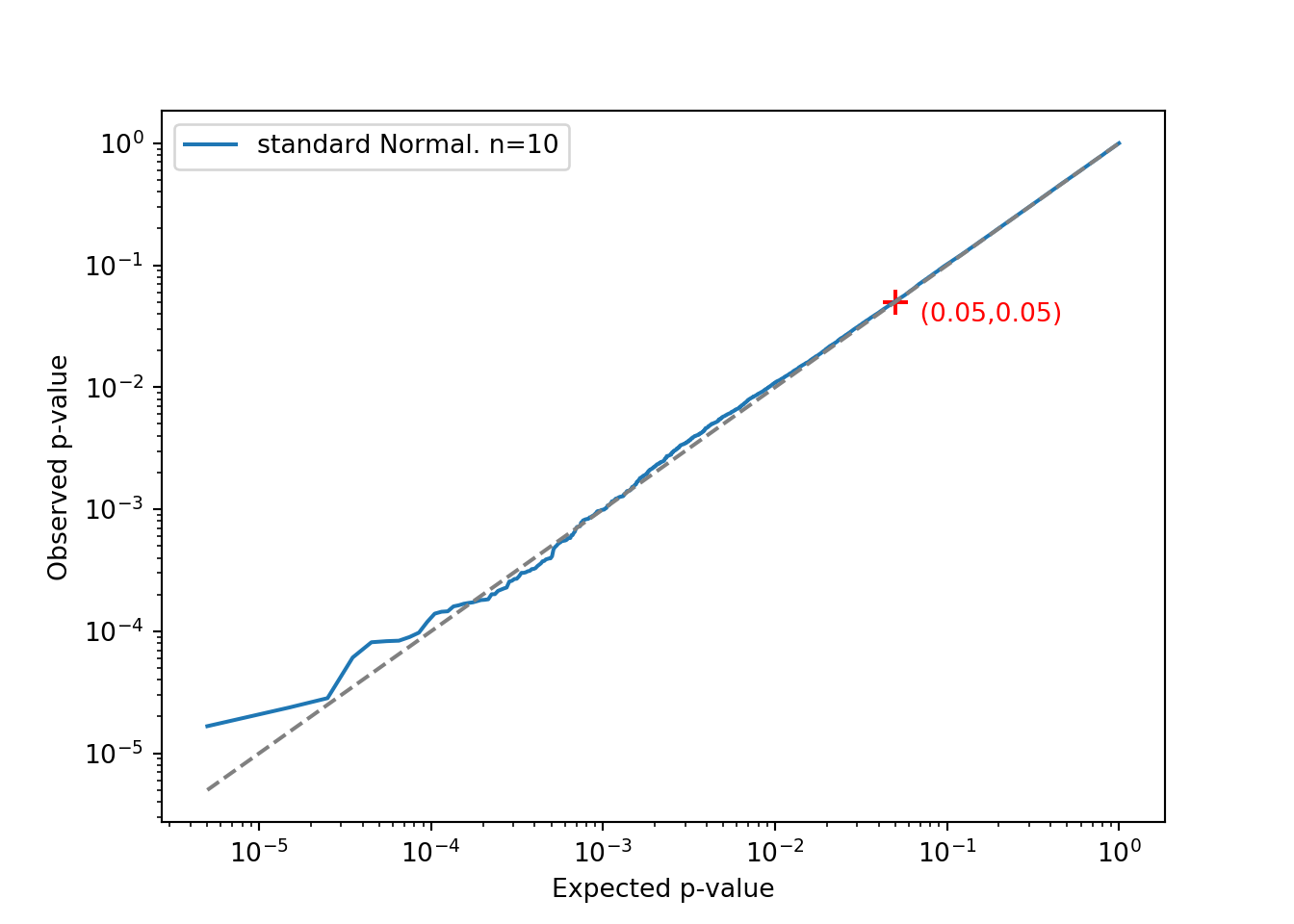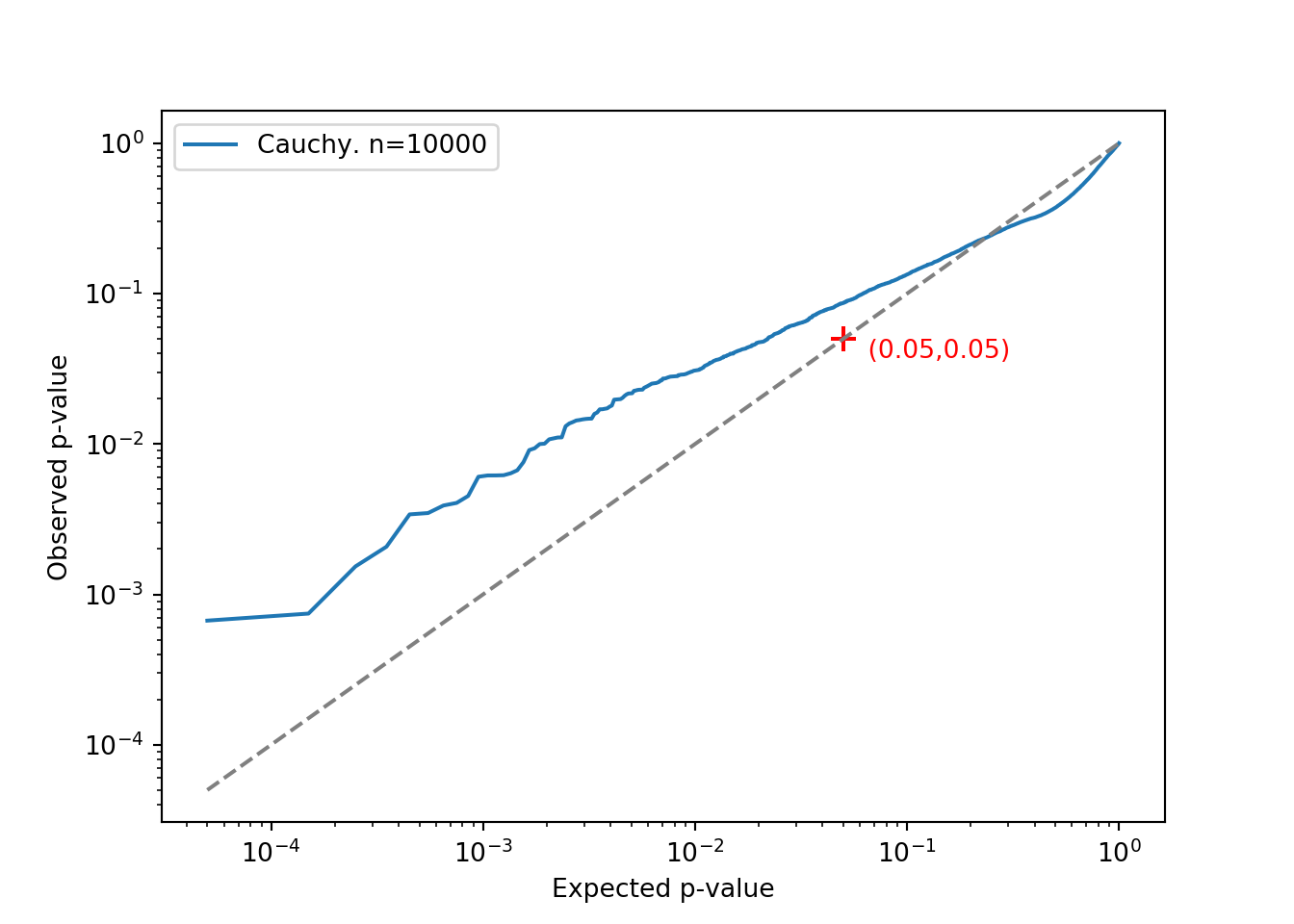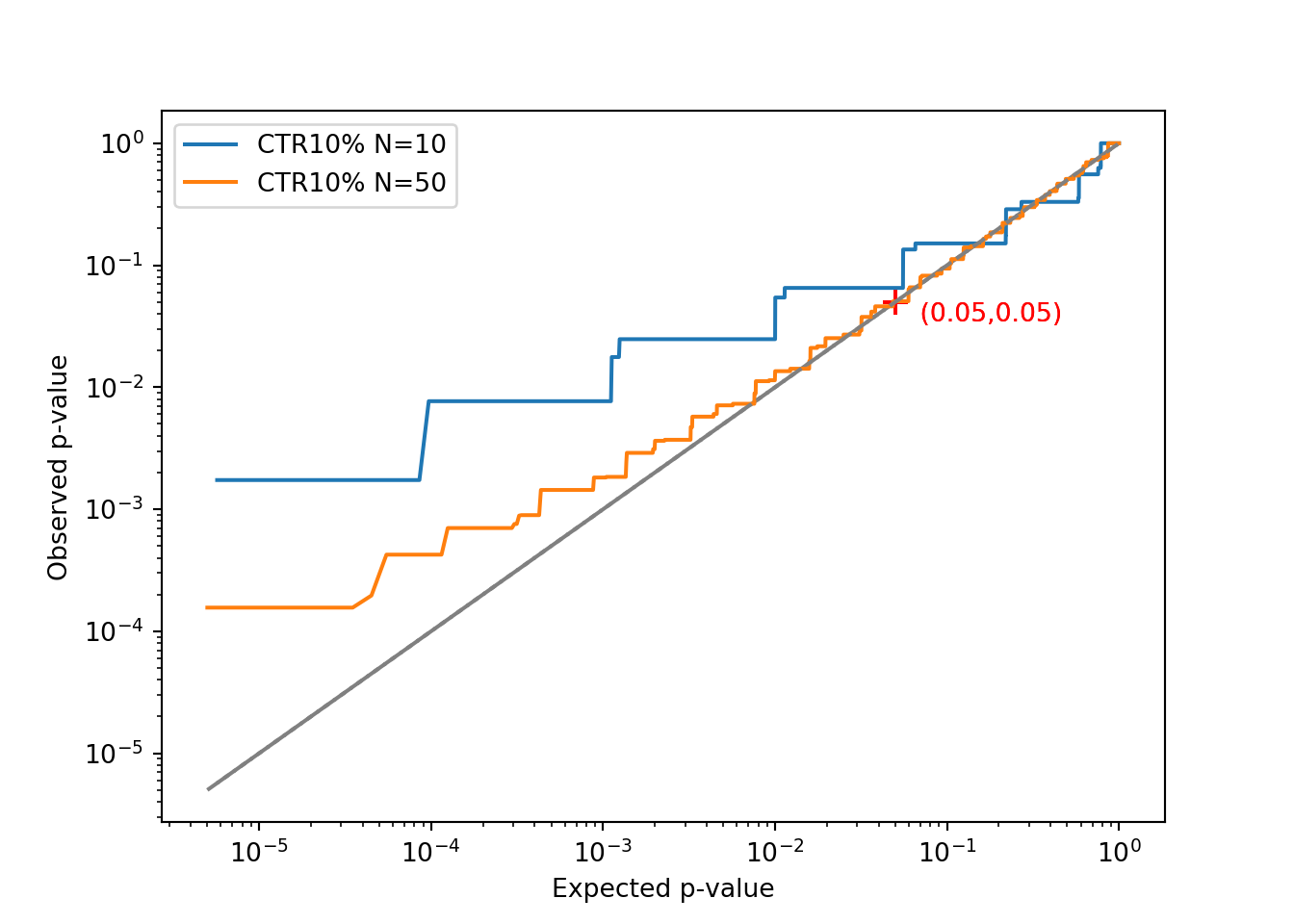# Aaron McDaid

Statistics and Python and C++, and other fun projects

aaron.mcdaid@gmail.com

@aaronmcdaid

by Aaron, 23rd July 2019

(source code to recreate this document)

Student’s t-test assumes normality in the data.

Well … I exaggerate a little. If your data is the revenue-per-order for example, the t-test does not require that the revenues be normally distributed. But it does require that the sample mean be normally distributed. In other words, if you select a sample of revenues at random and compute the mean of this sample, and if you repeat this procedure many times, the distribution of the sample mean must be approximately normal; this is the Central Limit Theorem.

But how large a sample is required? And how close to normal must the sample mean be?

This is quite a simplistic presentation in this blog - I would appreciate pointers to something more thorough and of higher quality!

## “The Large Enough Sample Condition”

It is sometimes said that thirty-to-fifty samples is enough. I’ve heard that many times, but I’ve never been satisfied with it. In this post, I’ll show that it’s not always a valid rule. I will simulate data from different distributions and investigate the limits of this rule.

First though, read the following quote, from here. It’s carefully presented and warns that a lot of assumptions need to be made before you can consider applying the thirty-to-fifty rule:

The Large Enough Sample Condition tests whether you have a large enough sample size compared to the population. A general rule of thumb for the Large Enough Sample Condition is that n≥30, where n is your sample size. However, it depends on what you are trying to accomplish and what you know about the distribution. In general, the Large Enough Sample Condition applies if any of these conditions are true:

• You have a symmetric distribution or unimodal distribution without outliers: a sample size of 15 is “large enough.”
• You have a moderately skewed distribution, that’s unimodal without outliers; If your sample size is between 16 and 40, it’s “large enough.”
• Your sample size is >40, as long as you do not have outliers.
• Your population has a normal distribution.

In the real world, for example in ecommerce applications, we often have very skewed distributions with a high rate of zeroes for example. And very tail-heavy with many extreme values. So none of these conditions hold

## How to simulate

First, some necessary imports.

import numpy as np
from scipy.stats import ttest_ind # t-test
import matplotlib
import matplotlib.pyplot as plt

We’ll need to draw two samples from the distribution of interest and then perform a t-test.

def draw_two_samples_and_do_a_ttest(src, n):
A = src(n)
B = src(n)
_, pvalue = ttest_ind(A, B)
return pvalue

… and we’ll need to perform this multiple times.

def repeatedly_draw_two_samples_and_do_a_ttest(src, n, M):
return [draw_two_samples_and_do_a_ttest(src, n) for _ in range(M)]

For example, using two samples from a normal distribution, with 100 samples in each. And repeating the process five times to get five pvalues:

standard_normal = lambda n: np.random.normal(size = n)
ps = repeatedly_draw_two_samples_and_do_a_ttest(standard_normal, 100, 5)
print(ps)
## [0.33936528943287825, 0.5735385762692617, 0.42613581472446016, 0.8056703527308852, 0.7576995127677106]

## Using qq-plots to evaluate the performance

If the null hypothesis is true, the pvalues will be uniformly distributed between 0 and 1. This is the criterion I will use here. If the pvalues deviate from this, then there is a problem with the assumptions.

In this plot, we simulate 100’000 t-tests and plot the 100’000 pvalues in ascending order. Note the log-log scale on this plot; this is done to allow us to focus on the smaller pvalues. A common $$\alpha$$ threshold is 5%, and therefore 5% of the pvalues should be less than 5%.

This 5% is marked as a small red cross in the plots. If the line of pvalues does not go through this point, then there is a problem.

We start with a very simple example. Drawing samples of size 10 from a Normal distribution. The line is very straight and goes through the red cross. So far, so good.

def qqplot(ys, label):
ys = sorted([y for y in ys if not np.isnan(y)])
N = len(ys)
xs = (np.arange(N)+0.5) / N
plt.loglog(xs, ys, label=label)
plt.xlabel("Expected p-value")
plt.ylabel("Observed p-value")
plt.plot([min(xs), 1], [min(xs), 1], color='grey', linestyle='dashed')
plt.scatter([0.05], [0.05], color='red', marker='+', s=100)
plt.text(0.05, 0.05, '   (0.05,0.05)', color='red', verticalalignment='top')
ps =  repeatedly_draw_two_samples_and_do_a_ttest(standard_normal, 10, 100000)
qqplot(ps, 'standard Normal. n=10')
plt.legend()
plt.show()## The Cauchy distribution

To go to an extreme, next I try the Cauchy distribution. Even with a large sample size (10’000) the distribution clearly deviates from uniformity.

plt.figure()
ps =  repeatedly_draw_two_samples_and_do_a_ttest(np.random.standard_cauchy, 10000, 10000)
qqplot(ps, 'Cauchy. n=10000')
plt.legend()
plt.show()This means the “thirty-to-fifty” rule doesn’t work if the underlying data is from a Cauchy distribution. In fact, even if you have fifty million points in your sample, the Central Limit Theorem will never apply to the Cauchy distribution. This is because the Cauchy does not have a finite variance.

## Outliers

I must say that I don’t quite know how to fit this observation into the “Large Enough Sample Condition” quote from the start of this article; the Cauchy distribution is symmetric and unimodal and isn’t skewed. I think the quote abuses the word “outlier” to imply that “without outliers” means the same as “has small variance”. I’ve never been satisfied with the general usage of the word “outlier”.

## Click-through rates

Anyway, you might be tempted to dismiss the Cauchy distribution as unrealistic. (It’s not!). But if you insist on something more realistic, let’s use the example of click-through rates instead.

If you are testing two different advertising strategies, you might compare the proportions of the ads that are clicked on. Let’s start with a very high click through rate of 10%.

With a very small sample size of 10, we see the pvalues are not uniform. Increasing the sample size to 50, we see that the pvalues become much more uniform in the vicinity of 5%, showing that the thirty-to-fifty rule works in this case:

click_through_rate_10 = lambda n: np.random.binomial(1, 0.10, n)
plt.figure()
ps =  repeatedly_draw_two_samples_and_do_a_ttest(click_through_rate_10, 10, 100000)
qqplot(ps, label='CTR10% N=10')
ps =  repeatedly_draw_two_samples_and_do_a_ttest(click_through_rate_10, 50, 100000)
qqplot(ps, label='CTR10% N=50')
plt.legend()
plt.show()But click through rates are much lower in reality: “Across all industries, the average CTR for a search ad is 1.91%, and 0.35% for a display ad.. We see here that 50 is not enough. Even with 200 samples, it still doesn’t look good:

click_through_rate_0035 = lambda n: np.random.binomial(1, 0.0035, n)
plt.figure()
for sample_size in [50, 200, 1000, 10000]:
ps =  repeatedly_draw_two_samples_and_do_a_ttest(click_through_rate_0035, sample_size, 10000)
qqplot(ps, label='CTR 0.35% N={}'.format(sample_size))
plt.legend()
plt.show()